# Understanding Multivariate Statistical Values (Advanced Signal Processing Toolkit)

LabVIEW 2014 Advanced Signal Processing Toolkit Help

Edition Date: June 2014

Part Number: 372656C-01

»View Product InfoDownload Help (Windows Only)

Multivariate statistical analysis methods enable you to investigate statistical interdependence between variables in a multivariate time series. These methods also help you perform blind source separation or eliminate redundant or extraneous variables in a multivariate time series. You also can use these methods to transform a multivariate time series so that information can be concentrated in a smaller number of variables, which enable you to reduce the dimensionality of multivariate time series.

## Understanding the Covariance Matrix

Covariance matrices measure the correlation between two or more time series acquired during the same period. In a unified covariance matrix or correlation-coefficient matrix, the diagonal values all have a value of one because all signals are correlated with themselves perfectly. Nondiagonal values close to one indicate that the corresponding variables are highly correlated.

Use the TSA Covariance VI to compute the covariance matrix and unified covariance matrix for multivariate time series.

## Understanding Principal Component Analysis

The main purpose of principal components analysis (PCA) is to enable you to isolate and remove extraneous or redundant variables in a multivariate time series. Extraneous and redundant variables increase the dimensionality of a time series and prevent you from finding important underlying patterns in the data. With the PCA method, you can reduce the dimensionality of a time series and retain as much information as possible. You also can make underlying patterns in the data more explicit and easier to find. PCA is useful in applications such as pattern recognition and image compression.

The following figure shows a simulated multivariate time series that contains two variables. These two variables are uncorrelated with each other.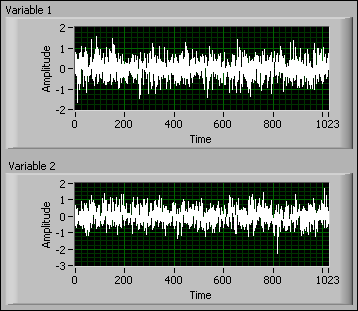The following figure shows the correlation and unified covariance matrix of the two variables. The Correlation graph is an XY graph that uses Variable 1 as x-axis and Variable 2 as y-axis. Variable 1 and Variable 2 are uncorrelated with each other because the data points spread across the XY graph irregularly. The nondiagonal elements in the unified Covariance Matrix are close to zero, so the two variables are uncorrelated.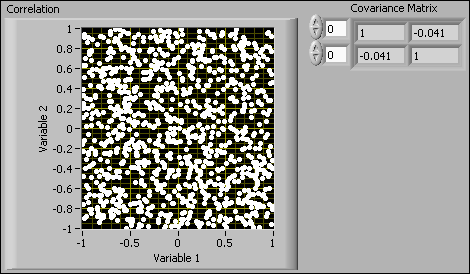The following figure shows a simulated multivariate time series that contains two variables. These two variables are correlated with each other.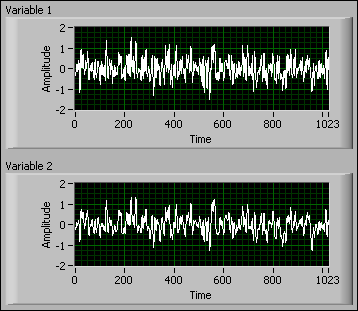The following figure shows the correlation and unified covariance matrix of the two variables. In the Correlation graph, Variable 1 and Variable 2 increase together. The nondiagonal elements in the unified Covariance Matrix are close to one, so the two variables are highly correlated.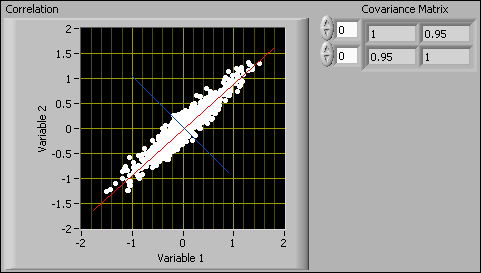PCA transforms correlated time series into uncorrelated time series. In the case of a two-variable time series, the first principal component is the line along the direction that has maximum variance. The second principal component is the line along the direction that has second largest variance and is perpendicular to the first principal component. In the previous figure, the red and blue lines represent the first principal component and the second principal component, respectively. The two lines form a new coordinate system. PCA rotates the original coordinate system to the new coordinate system and reduces the correlation between variables, potentially enabling you to eliminate the component with the lower variance if you judge the variance to be negligible in the application.

The following figure shows the correlation plot and unified covariance matrix of the resulting time series from PCA.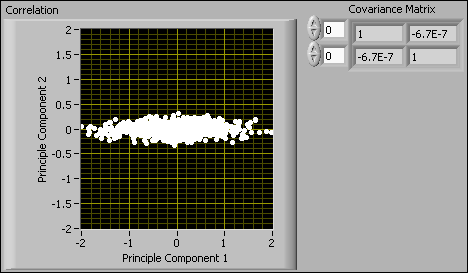PCA is not limited to two-variable time series. When the number of variables is greater than two, the eigenvectors of the correlation matrix are principal components. PCA reorders the eigenvectors based on the corresponding eigenvalues and projects the original time series on the eigenvectors. Principal component scores describe the projection results of the time series on the new coordinate system. Principal component scores are the linear combination of variables in the original time series.

The following figure is an example that uses PCA for image compression. The following figure shows an x-ray image. You can consider each row of the image as a time series and the whole image as a multivariate time series.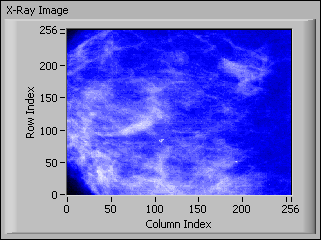You can check the correlation between each row of the image by computing the unified covariance matrix. The following figure shows part of the unified covariance matrix for the image in the previous figure. The nondiagonal elements are close to one. Therefore, strong correlation exists among some of the rows of the image. You can reduce the correlation by performing PCA on the original image.The following figure shows the PCA result of the image. The variance of each row decreases as the row index increase. The rows contain less significance as the row index increases. Deciding which rows are significant or useful is a matter of judgment, but a reasonable threshold is at row 50. If you keep only the first 50 rows, you can reduce the data dimensionality and corresponding image size in bits while retaining important information in the image.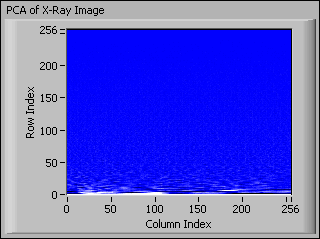The following figure shows the reconstructed image with the principal component scores of only the first 50 principal components. You can see that the reconstructed image properly retains the major features of the original image.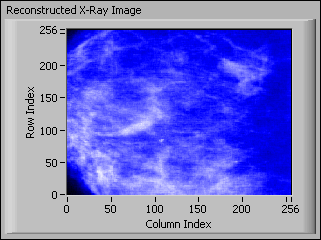Use the TSA Principal Component Analysis VI to perform PCA on multivariate time series.

Refer to the Image Compression with PCA VI in the labview\examples\Time Series Analysis\TSAApplications directory for an example that demonstrates an application of PCA in image compression.Note  The PCA technique is a linear transform. You cannot use PCA to process a multivariate time series that contains too much nonlinear correlation between variables.

## Understanding Independent Component Analysis

Independent component analysis (ICA) generates a multivariate time series with statistically-independent components from an original multivariate time series with statistically-dependent components. ICA is a generalization of PCA. ICA removes not only the second-order statistical dependency but also high-order statistical dependencies between the variables of a multivariate time series. However, PCA removes only the second-order statistical dependency between the variables.

One typical application of ICA is blind source separation, or revealing independent sources from sensor observations that are unknown linear mixtures of the unobserved source signals. The following figure illustrates the flowchart of blind source separation.In the previous figure, the observed signals are the linear mixtures of a set of unknown independent source signals. ICA estimates the source signals and the mixing matrix with only the observed signals. The estimated source signals are called independent components because they are statistically independent of each other.

For example, the electroencephalogram (EEG) data are recordings of electrical potentials at many different locations on a human scalp. These electrical potentials are the mixtures of signals generated by brain activities. ICA can help you recover the components of brain activities and reveal underlying information about those activities.

Another application of ICA is removing artifacts from signals. For example, in biomedical signal analysis, the magnetoencephalography (MEG) signals from a human brain usually contain artifacts such as eye movements, heartbeats, and measurement noise. You can use ICA to remove the artifacts and enhance the MEG signals. Using ICA to remove artifacts usually involves the following steps:

1. Compute the separating matrix, that is, the inverse of the mixing matrix, and obtain the independent components.
2. Remove undesirable independent components by setting their values to zeros.
3. Reconstruct signals from independent components with the mixing matrix.

You also can use ICA in the applications of feature extraction and data mining because ICA can make the features more explicit in the resulting independent components.

Use the TSA Independent Component Analysis VI to perform ICA on multivariate time series.

Refer to the Independent Component Analysis VI in the labview\examples\Time Series Analysis\TSAGettingStarted directory for an example that demonstrates how to perform independent component analysis on a multivariate time series.